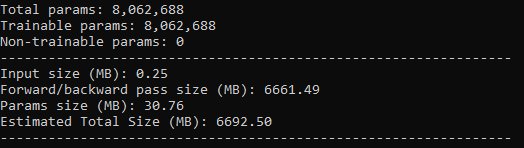# Cuda runs out of memory

I am building a custom CNN for image classification without a fully connected linear layer. The idea is to have 5 basic convolutional blocks (conv → relu → batch norm) then 12 residual blocks and finally 5 pooling layers to reduce the out shape to just one channel which will then go to a sigmoid layer for either 0 or 1.

However I keep getting this error that Cuda runs out of memory:

RuntimeError: CUDA out of memory. Tried to allocate 994.00 MiB (GPU 0; 11.91 GiB total capacity; 10.60 GiB already allocated; 750.94 MiB free; 10.63 GiB reserved in total by PyTorch)

Okay fair enough I drop the number of residuals to 7 then to 3 and I still get the same error for the same amount of memory that is 994.00 MiB even though obviously the number of trainable parameters are dropped drastically.

I got two questions. How come the error is over the same amount of memory? How come I run out of memory for a few layers when I can run densenet, inceptionv3, resnet largest variations on my other GPU, RTX 2080 11gb, which all have hundreds of layers with no problem at all?

I have provided code for the blocks of my network. The training process is the same and have been using it for ages for any generic model so that is not the issue at all.

``````     def __init__(self, in_features):
super(ResidualBlock, self).__init__()

nn.Conv2d(in_features, in_features, 3),
nn.BatchNorm2d(in_features),
nn.ReLU(inplace=True),
nn.Conv2d(in_features, in_features, 3),
nn.BatchNorm2d(in_features)  ]

self.conv_block = nn.Sequential(*conv_block)

def forward(self, x):
return x + self.conv_block(x)

class BasicConv2d(nn.Module):

def __init__(self, in_channels: int, out_channels: int, **kwargs: Any) -> None:
super(BasicConv2d, self).__init__()
self.conv = nn.Conv2d(in_channels, out_channels, bias=False, **kwargs)
self.bn = nn.BatchNorm2d(out_channels, eps=0.001)

def forward(self, x: Tensor) -> Tensor:
x = self.conv(x)
x = self.bn(x)
return F.relu(x, inplace=True)

class PoolingBlock(nn.Module):

def __init__(self, in_channels: int, out_channels: int, **kwargs: Any) -> None:
super(PoolingBlock, self).__init__()
self.conv = nn.Conv2d(in_channels, out_channels, bias=False, **kwargs)
self.bn = nn.BatchNorm2d(out_channels)
self.relu = nn.ReLU(inplace=True)
self.pooling = nn.MaxPool2d(2)

def forward(self, x: Tensor) -> Tensor:
x = self.conv(x)
x = self.bn(x)
x = self.relu(x)
return self.pooling(x)

class CustomNet(nn.Module):
def __init__(self):
super(CustomNet, self).__init__()
self.conv1 = BasicConv2d(1, 32, kernel_size=(3,3), padding=(3,3))
self.conv2 = BasicConv2d(32, 64, kernel_size=(3,3))
self.conv3 = BasicConv2d(64, 128, kernel_size=(3,3))
self.conv4 = BasicConv2d(128, 256, kernel_size=(3,3))
self.conv5 = BasicConv2d(256, 256, kernel_size=(3,3))
# self.conv6 = BasicConv2d(512, 512, kernel_size=(3,3))
# residuals
sequence = [ResidualBlock(256)]
for _ in range (2):
sequence += [ResidualBlock(256)]
self.residuals = nn.Sequential(*sequence)
# pooling layers
nfc = 256
pooling = [PoolingBlock(nfc, nfc // 2, kernel_size=(1, 1))]
for _ in range (6):
print(nfc)
nfc = nfc // 2
pooling += [PoolingBlock(nfc, nfc // 2, kernel_size=(1, 1))]
self.pool = nn.Sequential(*pooling)
self.fc = nn.Conv2d(2, 1, kernel_size=(1, 1), bias=False)

def forward(self, x):
x = self.conv1(x)
x = self.conv2(x)
x = self.conv3(x)
x = self.conv4(x)
x = self.conv5(x)
# x = self.conv6(x)
x = self.residuals(x)
x = self.pool(x)
x = self.fc(x)
return x
type or paste code here

The input to the network is 16 x 1 x 256 x 256

The memory problem occurs on the very first iteration once it hits the very first residual forward pass.``````

Model looks ok overall
I would run torchinfo to estimate number of parameters and model size firstI use torchsummary with summary(model, (1, 256, 256))

6.6Gb is a big one. During training, because of additional space required for graph, it’ll need much more than 11gb you have.

I would try to call model.float() before torchinfo() in case model is using float64.

I did model.float() and then torchinfo and i get the same size for forward pass